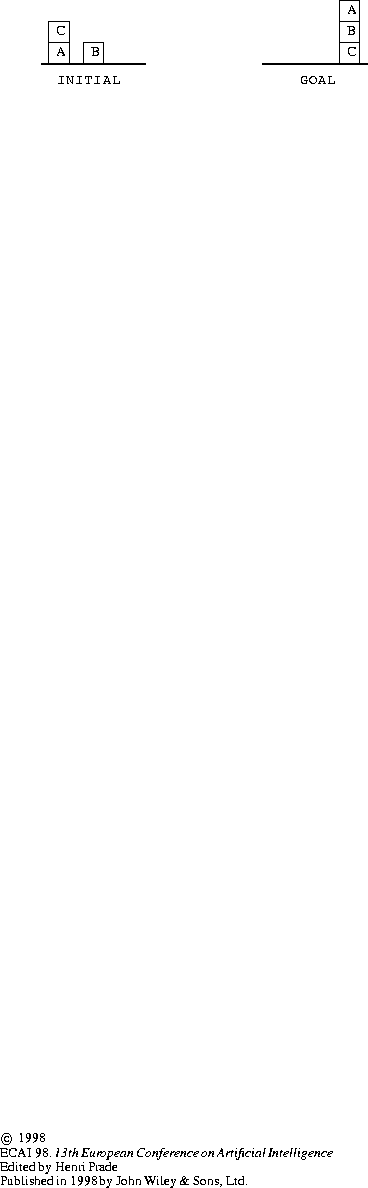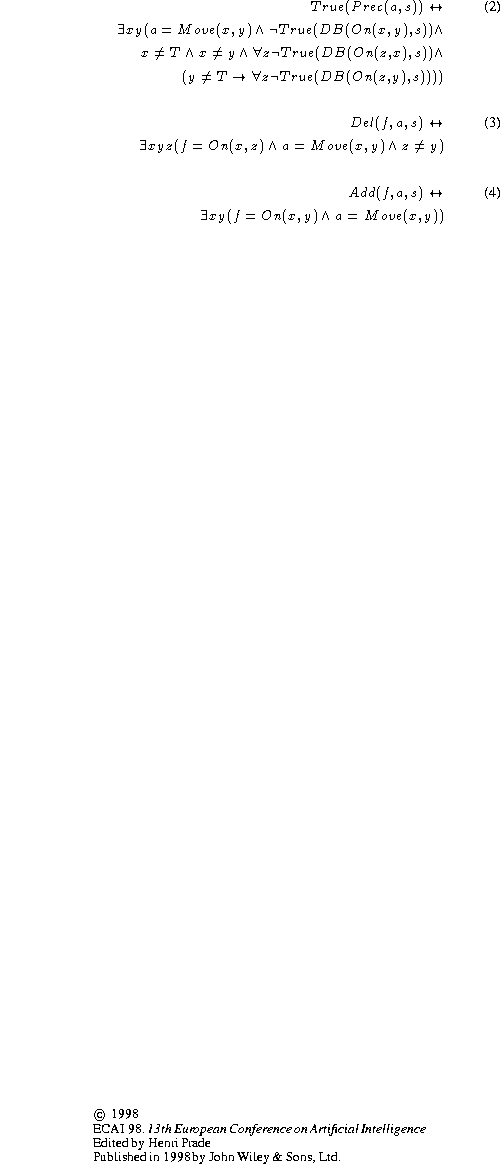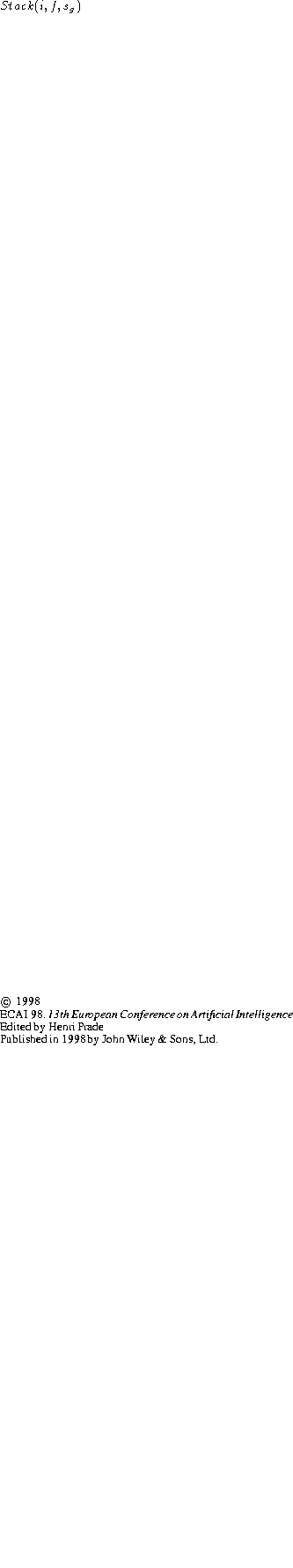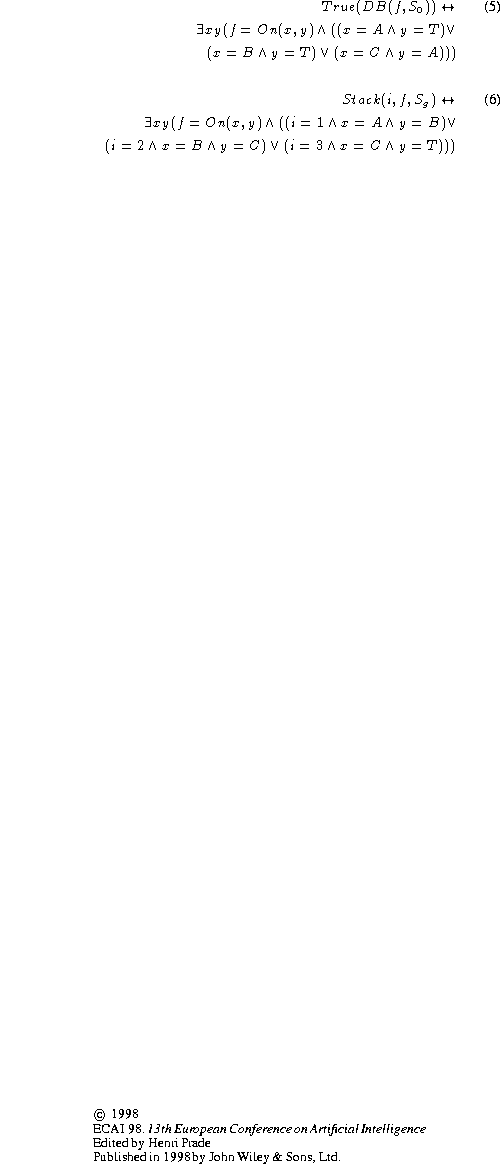Next: THE REASONING STRATEGY Up: THE REPRESENTATION OF PLANNING Previous: THE REPRESENTATION OF PLANNING

## Blocks World

As an example, we formalize Sussman's anomaly problem within this framework. The individual variables x, y and z range over blocks. The constant blocks are A, B, C and T (for Table). The function On maps a pair of blocks x and y into the reified formula of the sort database On(x,y) describing the fact that block x is on block y. The function Move maps a pair of blocks x and y into the action Move(x,y) denoting the act of moving block x on top of block y. The precondition, delete list, and add list of Move(x,y) are described as follows.Figure: Sussman's anomaly.The conditions on the initial and goal situations (and) are respectively described by axiomsand. The formulais true iff formula f is at location i in the stack describing.Josefina Sierra
Tue Jul 21 09:26:01 PDT 1998